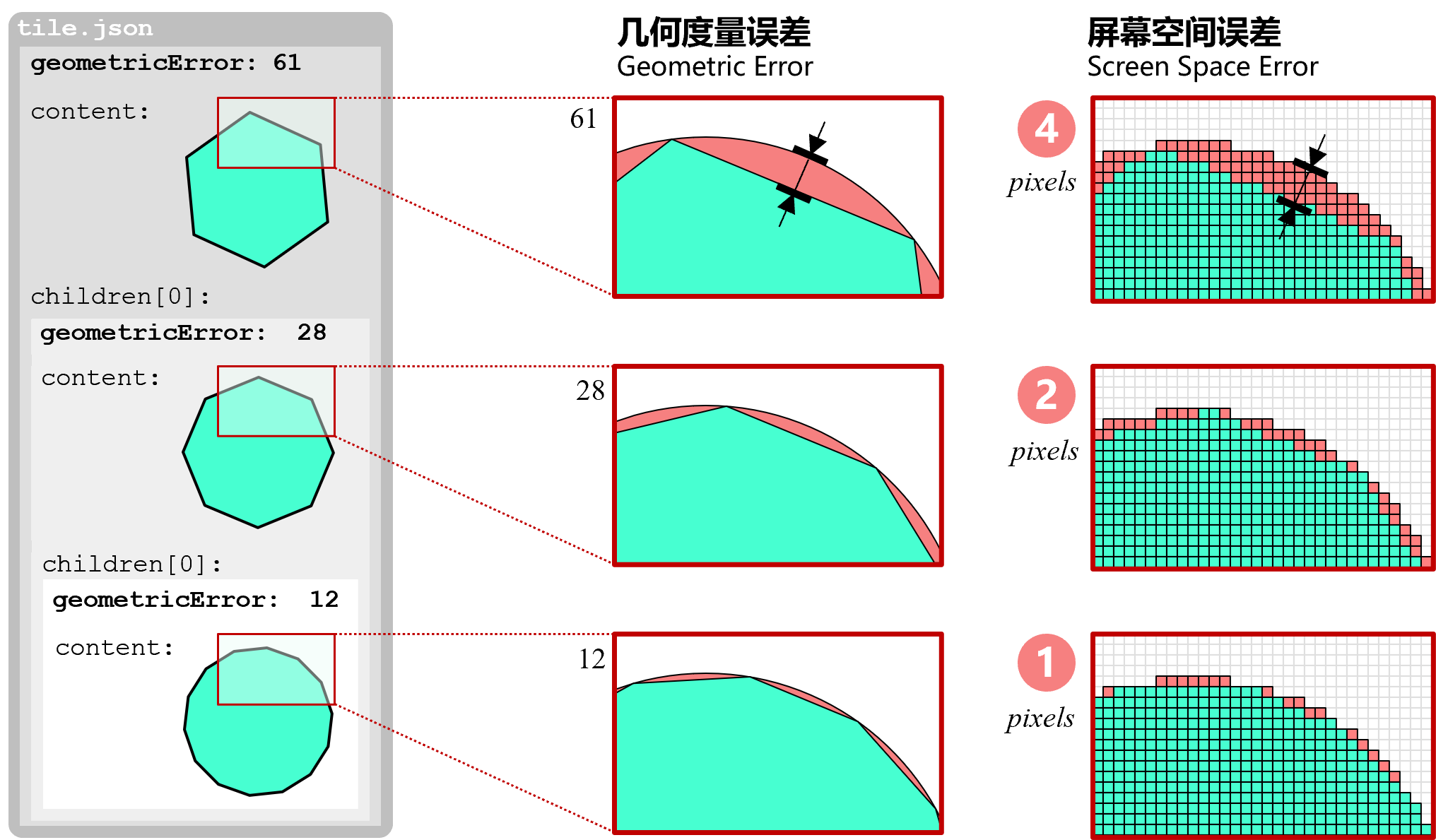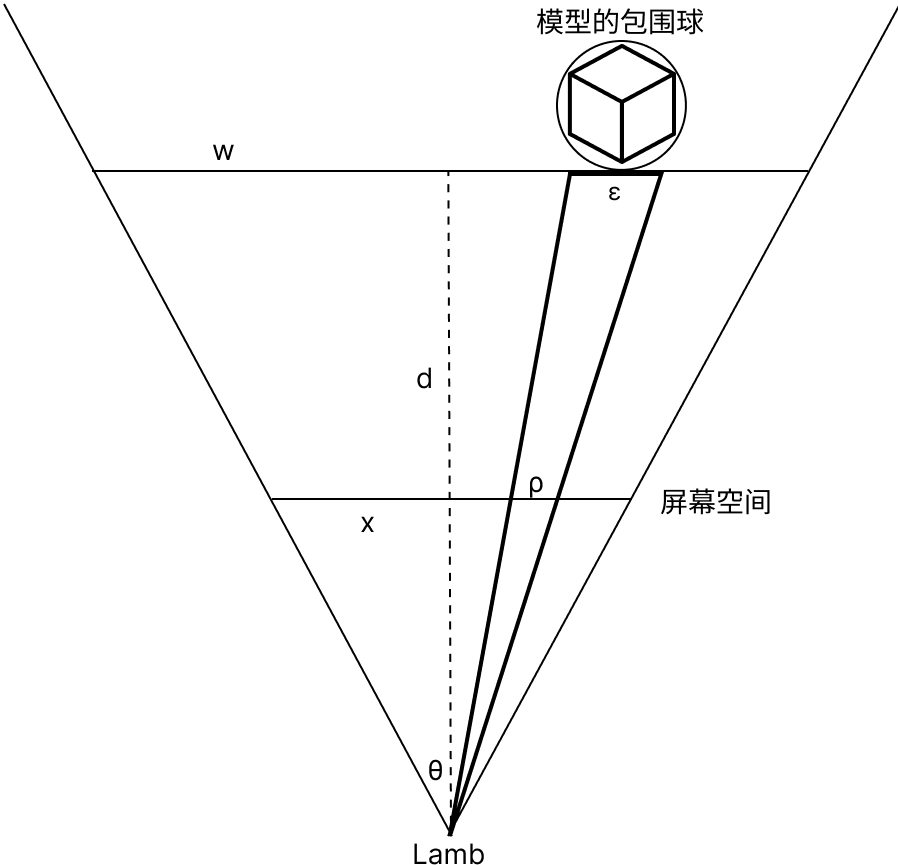## 概念## 计算$\frac{ε} {w} = \frac{ρ} {x}$

$w = 2dtan\frac{θ} {2}$

$d = (c - vierer) · v - r$

w 代入即可求得当前模型在当前状态下的 SSE :

$ρ = \frac{εx} {2dtan\frac{θ} {2}}$